xNewton's laws of motionOverview

Newton's laws of motion are three physical law
Physical law
A physical law or scientific law is "a theoretical principle deduced from particular facts, applicable to a defined group or class of phenomena, and expressible by the statement that a particular phenomenon always occurs if certain conditions be present." Physical laws are typically conclusions...

s that form the basis for classical mechanics
Classical mechanics
In physics, classical mechanics is one of the two major sub-fields of mechanics, which is concerned with the set of physical laws describing the motion of bodies under the action of a system of forces...

. They describe the relationship between the force
Force
In physics, a force is any influence that causes an object to undergo a change in speed, a change in direction, or a change in shape. In other words, a force is that which can cause an object with mass to change its velocity , i.e., to accelerate, or which can cause a flexible object to deform...

s acting on a body and its motion
Motion (physics)
In physics, motion is a change in position of an object with respect to time. Change in action is the result of an unbalanced force. Motion is typically described in terms of velocity, acceleration, displacement and time . An object's velocity cannot change unless it is acted upon by a force, as...

due to those forces. They have been expressed in several different ways over nearly three centuries, and can be summarized as follows:
1. First law: The velocity
Velocity
In physics, velocity is speed in a given direction. Speed describes only how fast an object is moving, whereas velocity gives both the speed and direction of the object's motion. To have a constant velocity, an object must have a constant speed and motion in a constant direction. Constant ...

of a body remains constant unless the body is acted upon by an external force.
2. Second law: The acceleration
Acceleration
In physics, acceleration is the rate of change of velocity with time. In one dimension, acceleration is the rate at which something speeds up or slows down. However, since velocity is a vector, acceleration describes the rate of change of both the magnitude and the direction of velocity. ...

a of a body is parallel
Parallel
-Mathematics and science:* Parallel , an imaginary east-west line circling a globe* Parallel circuits, as opposed to series* Parallel * Parallel evolution* Parallel transport* Parallel of declination, used in astronomy-Computing:...

and directly proportional to the net force
Force
In physics, a force is any influence that causes an object to undergo a change in speed, a change in direction, or a change in shape. In other words, a force is that which can cause an object with mass to change its velocity , i.e., to accelerate, or which can cause a flexible object to deform...

F and inversely proportional to the mass
Mass
Mass can be defined as a quantitive measure of the resistance an object has to change in its velocity.In physics, mass commonly refers to any of the following three properties of matter, which have been shown experimentally to be equivalent:...

m, i.e., F = ma.
3. Third law: The mutual forces of action and reaction between two bodies are equal, opposite and collinear.

The three laws of motion were first compiled by Sir Isaac Newton
Isaac Newton
Sir Isaac Newton PRS was an English physicist, mathematician, astronomer, natural philosopher, alchemist, and theologian, who has been "considered by many to be the greatest and most influential scientist who ever lived."...

in his work Philosophiæ Naturalis Principia Mathematica, first published on July 5, 1687.how does newtons laws of motion deal with the spport of basketball?DiscussionsWhat other phenomenonWere these laws published or were just passed down?Does NewtonWhat other laws did Newton come up?How many laws form NewtonEncyclopedia
Newton's laws of motion are three physical law
Physical law
A physical law or scientific law is "a theoretical principle deduced from particular facts, applicable to a defined group or class of phenomena, and expressible by the statement that a particular phenomenon always occurs if certain conditions be present." Physical laws are typically conclusions...

s that form the basis for classical mechanics
Classical mechanics
In physics, classical mechanics is one of the two major sub-fields of mechanics, which is concerned with the set of physical laws describing the motion of bodies under the action of a system of forces...

. They describe the relationship between the force
Force
In physics, a force is any influence that causes an object to undergo a change in speed, a change in direction, or a change in shape. In other words, a force is that which can cause an object with mass to change its velocity , i.e., to accelerate, or which can cause a flexible object to deform...

s acting on a body and its motion
Motion (physics)
In physics, motion is a change in position of an object with respect to time. Change in action is the result of an unbalanced force. Motion is typically described in terms of velocity, acceleration, displacement and time . An object's velocity cannot change unless it is acted upon by a force, as...

due to those forces. They have been expressed in several different ways over nearly three centuries, and can be summarized as follows:
1. First law: The velocity
Velocity
In physics, velocity is speed in a given direction. Speed describes only how fast an object is moving, whereas velocity gives both the speed and direction of the object's motion. To have a constant velocity, an object must have a constant speed and motion in a constant direction. Constant ...

of a body remains constant unless the body is acted upon by an external force.
2. Second law: The acceleration
Acceleration
In physics, acceleration is the rate of change of velocity with time. In one dimension, acceleration is the rate at which something speeds up or slows down. However, since velocity is a vector, acceleration describes the rate of change of both the magnitude and the direction of velocity. ...

a of a body is parallel
Parallel
-Mathematics and science:* Parallel , an imaginary east-west line circling a globe* Parallel circuits, as opposed to series* Parallel * Parallel evolution* Parallel transport* Parallel of declination, used in astronomy-Computing:...

and directly proportional to the net force
Force
In physics, a force is any influence that causes an object to undergo a change in speed, a change in direction, or a change in shape. In other words, a force is that which can cause an object with mass to change its velocity , i.e., to accelerate, or which can cause a flexible object to deform...

F and inversely proportional to the mass
Mass
Mass can be defined as a quantitive measure of the resistance an object has to change in its velocity.In physics, mass commonly refers to any of the following three properties of matter, which have been shown experimentally to be equivalent:...

m, i.e., F = ma.
3. Third law: The mutual forces of action and reaction between two bodies are equal, opposite and collinear.

The three laws of motion were first compiled by Sir Isaac Newton
Isaac Newton
Sir Isaac Newton PRS was an English physicist, mathematician, astronomer, natural philosopher, alchemist, and theologian, who has been "considered by many to be the greatest and most influential scientist who ever lived."...

in his work Philosophiæ Naturalis Principia Mathematica, first published on July 5, 1687. Newton used them to explain and investigate the motion of many physical objects and systems. For example, in the third volume of the text, Newton showed that these laws of motion, combined with his law of universal gravitation
Newton's law of universal gravitation
Newton's law of universal gravitation states that every point mass in the universe attracts every other point mass with a force that is directly proportional to the product of their masses and inversely proportional to the square of the distance between them...

, explained Kepler's laws of planetary motion
Kepler's laws of planetary motion
In astronomy, Kepler's laws give a description of the motion of planets around the Sun.Kepler's laws are:#The orbit of every planet is an ellipse with the Sun at one of the two foci....

.

## Overview

Newton's laws are applied to bodies (objects) which are considered or idealized as a particle, in the sense that the extent of the body is neglected in the evaluation of its motion, i.e., the object is small compared to the distances involved in the analysis, or the deformation
Deformation (mechanics)
Deformation in continuum mechanics is the transformation of a body from a reference configuration to a current configuration. A configuration is a set containing the positions of all particles of the body...

and rotation of the body is of no importance in the analysis. Therefore, a planet can be idealized as a particle for analysis of its orbital motion around a star.

In their original form, Newton's laws of motion are not adequate to characterize the motion of rigid bodies and deformable bodies. Leonard Euler in 1750 introduced a generalization of Newton's laws of motion for rigid bodies called the Euler's laws of motion, later applied as well for deformable bodies assumed as a continuum
Continuum mechanics
Continuum mechanics is a branch of mechanics that deals with the analysis of the kinematics and the mechanical behavior of materials modelled as a continuous mass rather than as discrete particles...

. If a body is represented as an assemblage of discrete particles, each governed by Newton’s laws of motion, then Euler’s laws can be derived from Newton’s laws. Euler’s laws can, however, be taken as axioms describing the laws of motion for extended bodies, independently of any particle structure.

Newton's Laws hold only with respect to a certain set of frames of reference
Frames of Reference
Frames of Reference is a 1960 educational film by Physical Sciences Study Committee.The film was made to be shown in high school physics courses. In the film University of Toronto physics professors Patterson Hume and Donald Ivey explain the distinction between inertial and nonintertial frames of...

called Newtonian or inertial reference frames. Some authors interpret the first law as defining what an inertial reference frame is; from this point of view, the second law only holds when the observation is made from an inertial reference frame, and therefore the first law cannot be proved as a special case of the second. Other authors do treat the first law as a corollary of the second. The explicit concept of an inertial frame of reference was not developed until long after Newton's death.

In the given interpretation mass, acceleration, momentum, and (most importantly) force are assumed to be externally defined quantities. This is the most common, but not the only interpretation: one can consider the laws to be a definition of these quantities.

At speeds approaching the speed of light
Speed of light
The speed of light in vacuum, usually denoted by c, is a physical constant important in many areas of physics. Its value is 299,792,458 metres per second, a figure that is exact since the length of the metre is defined from this constant and the international standard for time...

the effects of special relativity
Special relativity
Special relativity is the physical theory of measurement in an inertial frame of reference proposed in 1905 by Albert Einstein in the paper "On the Electrodynamics of Moving Bodies".It generalizes Galileo's...

must be taken into account.

## Newton's first law

This law states that if the resultant force (the vector sum of all forces acting on an object) is zero, then the velocity
Velocity
In physics, velocity is speed in a given direction. Speed describes only how fast an object is moving, whereas velocity gives both the speed and direction of the object's motion. To have a constant velocity, an object must have a constant speed and motion in a constant direction. Constant ...

of the object is constant. Mathematically stated: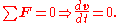Consequently:
• An object that is at rest will stay at rest unless an unbalanced force acts upon it.
• An object that is in motion will not change its velocity unless an unbalanced force acts upon it.

Newton placed the first law of motion to establish frames of reference
Frames of Reference
Frames of Reference is a 1960 educational film by Physical Sciences Study Committee.The film was made to be shown in high school physics courses. In the film University of Toronto physics professors Patterson Hume and Donald Ivey explain the distinction between inertial and nonintertial frames of...

for which the other laws are applicable. The first law of motion postulates the existence of at least one frame of reference
Frame of reference
A frame of reference in physics, may refer to a coordinate system or set of axes within which to measure the position, orientation, and other properties of objects in it, or it may refer to an observational reference frame tied to the state of motion of an observer.It may also refer to both an...

called a Newtonian or inertial reference frame, relative to which the motion of a particle not subject to forces is a straight line at a constant speed. Newton's first law is often referred to as the law of inertia
Inertia
Inertia is the resistance of any physical object to a change in its state of motion or rest, or the tendency of an object to resist any change in its motion. It is proportional to an object's mass. The principle of inertia is one of the fundamental principles of classical physics which are used to...

. Thus, a condition necessary for the uniform motion of a particle relative to an inertial reference frame is that the total net force
Force
In physics, a force is any influence that causes an object to undergo a change in speed, a change in direction, or a change in shape. In other words, a force is that which can cause an object with mass to change its velocity , i.e., to accelerate, or which can cause a flexible object to deform...

acting on it is zero. In this sense, the first law can be restated as:
Newton's laws are valid only in an inertial reference frame. Any reference frame that is in uniform motion with respect to an inertial frame is also an inertial frame, i.e. Galilean invariance
Galilean invariance
Galilean invariance or Galilean relativity is a principle of relativity which states that the fundamental laws of physics are the same in all inertial frames...

or the principle of Newtonian relativity
Principle of relativity
In physics, the principle of relativity is the requirement that the equations describing the laws of physics have the same form in all admissible frames of reference....

.

Newton's first law is a restatement of the law of inertia which Galileo had already described and Newton gave credit to Galileo. Aristotle
Aristotle
Aristotle was a Greek philosopher and polymath, a student of Plato and teacher of Alexander the Great. His writings cover many subjects, including physics, metaphysics, poetry, theater, music, logic, rhetoric, linguistics, politics, government, ethics, biology, and zoology...

had the view that all objects have a natural place in the universe: that heavy objects like rocks wanted to be at rest on the Earth and that light objects like smoke wanted to be at rest in the sky and the stars wanted to remain in the heavens. He thought that a body was in its natural state when it was at rest, and for the body to move in a straight line at a constant speed an external agent was needed to continually propel it, otherwise it would stop moving. Galileo, however, realized that a force is necessary to change the velocity of a body, i.e., acceleration, but no force is needed to maintain its velocity. This insight leads to Newton's First Law —no force means no acceleration, and hence the body will maintain its velocity.

The law of inertia apparently occurred to several different natural philosophers and scientists independently. The 17th century philosopher René Descartes
René Descartes
René Descartes ; was a French philosopher and writer who spent most of his adult life in the Dutch Republic. He has been dubbed the 'Father of Modern Philosophy', and much subsequent Western philosophy is a response to his writings, which are studied closely to this day...

also formulated the law, although he did not perform any experiments to confirm it.

## Newton's second law

The second law states that the net force
Net force
In physics, net force is the total force acting on an object. It is calculated by vector addition of all forces that are actually acting on that object. Net force has the same effect on the translational motion of the object as all actual forces taken together...

on a particle is equal to the time rate of change of its linear momentum
Momentum
In classical mechanics, linear momentum or translational momentum is the product of the mass and velocity of an object...

p in an inertial reference frame: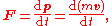where, since the law is valid only for constant-mass systems, the mass can be taken outside the differentiation
Differentiation
Differentiation may refer to:* Differentiation , the process of finding a derivative* Differentiated instruction in education* Cellular differentiation in biology* Planetary differentiation in planetary science...

operator by the constant factor rule in differentiation
Constant factor rule in differentiation
In calculus, the constant factor rule in differentiation, also known as The Kutz Rule, allows you to take constants outside a derivative and concentrate on differentiating the function of x itself...

. Thus,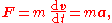where F is the net force applied, m is the mass of the body, and a is the body's acceleration. Thus, the net force applied to a body produces a proportional acceleration. In other words, if a body is accelerating, then there is a force on it.

Any mass that is gained or lost by the system will cause a change in momentum that is not the result of an external force. A different equation is necessary for variable-mass systems (see below).

Consistent with the first law, the time derivative of the momentum is non-zero when the momentum changes direction, even if there is no change in its magnitude; such is the case with uniform circular motion
Uniform circular motion
In physics, uniform circular motion describes the motion of a body traversing a circular path at constant speed. The distance of the body from the axis of rotation remains constant at all times. Though the body's speed is constant, its velocity is not constant: velocity, a vector quantity, depends...

. The relationship also implies the conservation of momentum: when the net force on the body is zero, the momentum of the body is constant. Any net force is equal to the rate of change of the momentum.

Newton's second law requires modification if the effects of special relativity
Special relativity
Special relativity is the physical theory of measurement in an inertial frame of reference proposed in 1905 by Albert Einstein in the paper "On the Electrodynamics of Moving Bodies".It generalizes Galileo's...

are to be taken into account, because at high speeds the approximation that momentum is the product of rest mass and velocity is not accurate.

### Impulse

An impulse J occurs when a force F acts over an interval of time Δt, and it is given by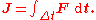Since force is the time derivative of momentum, it follows that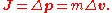This relation between impulse and momentum is closer to Newton's wording of the second law.

Impulse is a concept frequently used in the analysis of collisions and impacts.

### Variable-mass systems

Variable-mass systems, like a rocket burning fuel and ejecting spent gases, are not closed
Closed system
-In physics:In thermodynamics, a closed system can exchange energy , but not matter, with its surroundings.In contrast, an isolated system cannot exchange any of heat, work, or matter with the surroundings, while an open system can exchange all of heat, work and matter.For a simple system, with...

and cannot be directly treated by making mass a function of time in the second law.

The reasoning, given in An Introduction to Mechanics by Kleppner and Kolenkow and other modern texts, is that Newton's second law applies fundamentally to particles. In classical mechanics, particles by definition have constant mass. In case of a well-defined system of particles, Newton's law can be extended by summing over all the particles in the system: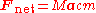where Fnet is the total external force on the system, M is the total mass of the system, and acm is the acceleration of the center of mass
Center of mass
In physics, the center of mass or barycenter of a system is the average location of all of its mass. In the case of a rigid body, the position of the center of mass is fixed in relation to the body...

of the system.

Variable-mass systems like a rocket or a leaking bucket cannot usually be treated as a system of particles, and thus Newton's second law cannot be applied directly. Instead, the general equation of motion for a body whose mass m varies with time by either ejecting or accreting mass is obtained by rearranging the second law and adding a term to account for the momentum carried by mass entering or leaving the system: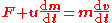where u is the relative velocity of the escaping or incoming mass with respect to the center of mass of the body. Under some conventions, the quantity (u dm/dt) on the left-hand side, known as the thrust
Thrust
Thrust is a reaction force described quantitatively by Newton's second and third laws. When a system expels or accelerates mass in one direction the accelerated mass will cause a force of equal magnitude but opposite direction on that system....

, is defined as a force (the force exerted on the body by the changing mass, such as rocket exhaust) and is included in the quantity F. Then, by substituting the definition of acceleration, the equation becomes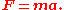### History

This was translated quite closely in Motte's 1729 translation as:
According to modern ideas of how Newton was using his terminology, this is understood, in modern terms, as an equivalent of:
Motte's 1729 translation of Newton's Latin continued with Newton's commentary on the second law of motion, reading:
The sense or senses in which Newton used his terminology, and how he understood the second law and intended it to be understood, have been extensively discussed by historians of science, along with the relations between Newton's formulation and modern formulations.

## Newton's third lawA more direct translation than the one just given above is:
In the above, as usual, motion is Newton's name for momentum, hence his careful distinction between motion and velocity.

The Third Law means that all forces are interactions between different bodies, and thus that there is no such thing as a unidirectional force or a force that acts on only one body. Whenever a first body exerts a force F on a second body, the second body exerts a force −F on the first body. F and −F are equal in magnitude and opposite in direction. This law is sometimes referred to as the action-reaction law
Reaction (physics)
The third of Newton's laws of motion of classical mechanics states that forces always occur in pairs. Every action is accompanied by a reaction of equal magnitude but opposite direction. This principle is commonly known in the Latin language as actio et reactio. The attribution of which of the two...

, with F called the "action" and −F the "reaction". The action and the reaction are simultaneous.

As shown in the diagram opposite, the skaters' forces on each other are equal in magnitude, but act in opposite directions. Although the forces are equal, the accelerations are not: the less massive skater will have a greater acceleration due to Newton's second law. The two forces in Newton's third law are of the same type (e.g., if the road exerts a forward frictional force on an accelerating car's tires, then it is also a frictional force that Newton's third law predicts for the tires pushing backward on the road).

Put very simply: a force acts between a pair of objects, and not on a single object. So each and every force has two ends. Each of the two ends is the same except for being opposite in direction. The ends of a force are mirror images of each other, one might say.

From a mathematical point of view, Newton's third law is a one-dimensional vector equation, which can be stated as follows. Given two objects A and B, each exerting a force on the other,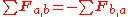where
Fa,b are the forces from B acting on A, and
Fb,a are the forces from A acting on B.

Newton used the third law to derive the law of conservation of momentum; however from a deeper perspective, conservation of momentum is the more fundamental idea (derived via Noether's theorem
Noether's theorem
Noether's theorem states that any differentiable symmetry of the action of a physical system has a corresponding conservation law. The theorem was proved by German mathematician Emmy Noether in 1915 and published in 1918...

from Galilean invariance
Galilean invariance
Galilean invariance or Galilean relativity is a principle of relativity which states that the fundamental laws of physics are the same in all inertial frames...

), and holds in cases where Newton's third law appears to fail, for instance when force fields as well as particles carry momentum, and in quantum mechanics
Quantum mechanics
Quantum mechanics, also known as quantum physics or quantum theory, is a branch of physics providing a mathematical description of much of the dual particle-like and wave-like behavior and interactions of energy and matter. It departs from classical mechanics primarily at the atomic and subatomic...

.

## Importance and range of validity

Newton's laws were verified by experiment and observation for over 200 years, and they are excellent approximations at the scales and speeds of everyday life. Newton's laws of motion, together with his law of universal gravitation and the mathematical techniques of calculus
Calculus
Calculus is a branch of mathematics focused on limits, functions, derivatives, integrals, and infinite series. This subject constitutes a major part of modern mathematics education. It has two major branches, differential calculus and integral calculus, which are related by the fundamental theorem...

, provided for the first time a unified quantitative explanation for a wide range of physical phenomena.

These three laws hold to a good approximation for macroscopic objects under everyday conditions. However, Newton's laws (combined with universal gravitation and classical electrodynamics) are inappropriate for use in certain circumstances, most notably at very small scales, very high speeds (in special relativity
Special relativity
Special relativity is the physical theory of measurement in an inertial frame of reference proposed in 1905 by Albert Einstein in the paper "On the Electrodynamics of Moving Bodies".It generalizes Galileo's...

, the Lorentz factor
Lorentz factor
The Lorentz factor or Lorentz term appears in several equations in special relativity, including time dilation, length contraction, and the relativistic mass formula. Because of its ubiquity, physicists generally represent it with the shorthand symbol γ . It gets its name from its earlier...

must be included in the expression for momentum along with rest mass and velocity) or very strong gravitational fields. Therefore, the laws cannot be used to explain phenomena such as conduction of electricity in a semiconductor
Semiconductor
A semiconductor is a material with electrical conductivity due to electron flow intermediate in magnitude between that of a conductor and an insulator. This means a conductivity roughly in the range of 103 to 10−8 siemens per centimeter...

, optical properties of substances, errors in non-relativistically corrected GPS systems and superconductivity
Superconductivity
Superconductivity is a phenomenon of exactly zero electrical resistance occurring in certain materials below a characteristic temperature. It was discovered by Heike Kamerlingh Onnes on April 8, 1911 in Leiden. Like ferromagnetism and atomic spectral lines, superconductivity is a quantum...

. Explanation of these phenomena requires more sophisticated physical theories, including general relativity
General relativity
General relativity or the general theory of relativity is the geometric theory of gravitation published by Albert Einstein in 1916. It is the current description of gravitation in modern physics...

and quantum field theory
Quantum field theory
Quantum field theory provides a theoretical framework for constructing quantum mechanical models of systems classically parametrized by an infinite number of dynamical degrees of freedom, that is, fields and many-body systems. It is the natural and quantitative language of particle physics and...

.

In quantum mechanics
Quantum mechanics
Quantum mechanics, also known as quantum physics or quantum theory, is a branch of physics providing a mathematical description of much of the dual particle-like and wave-like behavior and interactions of energy and matter. It departs from classical mechanics primarily at the atomic and subatomic...

concepts such as force, momentum, and position are defined by linear operators
Operator (physics)
In physics, an operator is a function acting on the space of physical states. As a resultof its application on a physical state, another physical state is obtained, very often along withsome extra relevant information....

that operate on the quantum state; at speeds that are much lower than the speed of light, Newton's laws are just as exact for these operators as they are for classical objects. At speeds comparable to the speed of light, the second law holds in the original form F = , which says that the force is the derivative of the momentum of the object with respect to time, but some of the newer versions of the second law (such as the constant mass approximation above) do not hold at relativistic velocities.

## Relationship to the conservation laws

In modern physics, the laws of conservation
Conservation law
In physics, a conservation law states that a particular measurable property of an isolated physical system does not change as the system evolves....

of momentum
Momentum
In classical mechanics, linear momentum or translational momentum is the product of the mass and velocity of an object...

, energy
Energy
In physics, energy is an indirectly observed quantity. It is often understood as the ability a physical system has to do work on other physical systems...

, and angular momentum
Angular momentum
In physics, angular momentum, moment of momentum, or rotational momentum is a conserved vector quantity that can be used to describe the overall state of a physical system...

are of more general validity than Newton's laws, since they apply to both light and matter, and to both classical and non-classical physics.

This can be stated simply, "Momentum, energy and angular momentum cannot be created or destroyed."

Because force is the time derivative of momentum, the concept of force is redundant and subordinate to the conservation of momentum, and is not used in fundamental theories (e.g., quantum mechanics
Quantum mechanics
Quantum mechanics, also known as quantum physics or quantum theory, is a branch of physics providing a mathematical description of much of the dual particle-like and wave-like behavior and interactions of energy and matter. It departs from classical mechanics primarily at the atomic and subatomic...

, quantum electrodynamics
Quantum electrodynamics
Quantum electrodynamics is the relativistic quantum field theory of electrodynamics. In essence, it describes how light and matter interact and is the first theory where full agreement between quantum mechanics and special relativity is achieved...

, general relativity
General relativity
General relativity or the general theory of relativity is the geometric theory of gravitation published by Albert Einstein in 1916. It is the current description of gravitation in modern physics...

, etc.). The standard model
Standard Model
The Standard Model of particle physics is a theory concerning the electromagnetic, weak, and strong nuclear interactions, which mediate the dynamics of the known subatomic particles. Developed throughout the mid to late 20th century, the current formulation was finalized in the mid 1970s upon...

explains in detail how the three fundamental forces known as gauge forces
Gauge theory
In physics, gauge invariance is the property of a field theory in which different configurations of the underlying fundamental but unobservable fields result in identical observable quantities. A theory with such a property is called a gauge theory...

originate out of exchange by virtual particles. Other forces such as gravity
Gravitation
Gravitation, or gravity, is a natural phenomenon by which physical bodies attract with a force proportional to their mass. Gravitation is most familiar as the agent that gives weight to objects with mass and causes them to fall to the ground when dropped...

and fermionic degeneracy pressure also arise from the momentum conservation. Indeed, the conservation of 4-momentum in inertial motion via curved space-time results in what we call gravitational force in general relativity
General relativity
General relativity or the general theory of relativity is the geometric theory of gravitation published by Albert Einstein in 1916. It is the current description of gravitation in modern physics...

theory. Application of space derivative (which is a momentum operator
Momentum operator
In quantum mechanics, momentum is defined as an operator on the wave function. The Heisenberg uncertainty principle defines limits on how accurately the momentum and position of a single observable system can be known at once...

in quantum mechanics) to overlapping wave functions of pair of fermion
Fermion
In particle physics, a fermion is any particle which obeys the Fermi–Dirac statistics . Fermions contrast with bosons which obey Bose–Einstein statistics....

s (particles with semi-integer spin
Spin (physics)
In quantum mechanics and particle physics, spin is a fundamental characteristic property of elementary particles, composite particles , and atomic nuclei.It is worth noting that the intrinsic property of subatomic particles called spin and discussed in this article, is related in some small ways,...

) results in shifts of maxima of compound wavefunction away from each other, which is observable as "repulsion" of fermions.

Newton stated the third law within a world-view that assumed instantaneous action at a distance between material particles. However, he was prepared for philosophical criticism of this action at a distance
Action at a distance (physics)
In physics, action at a distance is the interaction of two objects which are separated in space with no known mediator of the interaction. This term was used most often in the context of early theories of gravity and electromagnetism to describe how an object responds to the influence of distant...

, and it was in this context that he stated the famous phrase "I feign no hypotheses
Hypotheses non fingo
Hypotheses non fingo is a famous phrase used by Isaac Newton in an essay General Scholium which was appended to the second edition of the Principia....

". In modern physics, action at a distance has been completely eliminated, except for subtle effects involving quantum entanglement
Quantum entanglement
Quantum entanglement occurs when electrons, molecules even as large as "buckyballs", photons, etc., interact physically and then become separated; the type of interaction is such that each resulting member of a pair is properly described by the same quantum mechanical description , which is...

. However in modern engineering in all practical applications involving the motion of vehicles and satellites, the concept of action at a distance is used extensively.

Conservation of energy
Conservation of energy
The nineteenth century law of conservation of energy is a law of physics. It states that the total amount of energy in an isolated system remains constant over time. The total energy is said to be conserved over time...

was discovered nearly two centuries after Newton's lifetime, the long delay occurring because of the difficulty in understanding the role of microscopic and invisible forms of energy such as heat and infra-red light.

• List of scientific laws named after people
• Newton's law of universal gravitation
Newton's law of universal gravitation
Newton's law of universal gravitation states that every point mass in the universe attracts every other point mass with a force that is directly proportional to the product of their masses and inversely proportional to the square of the distance between them...

• Mercury, orbit of
• Galilean invariance
Galilean invariance
Galilean invariance or Galilean relativity is a principle of relativity which states that the fundamental laws of physics are the same in all inertial frames...

• Modified Newtonian dynamics
Modified Newtonian dynamics
In physics, Modified Newtonian dynamics is a hypothesis that proposes a modification of Newton's law of gravity to explain the galaxy rotation problem. When the uniform velocity of rotation of galaxies was first observed, it was unexpected because Newtonian theory of gravity predicts that objects...

• Lagrangian mechanics
Lagrangian mechanics
Lagrangian mechanics is a re-formulation of classical mechanics that combines conservation of momentum with conservation of energy. It was introduced by the Italian-French mathematician Joseph-Louis Lagrange in 1788....

• Hamiltonian mechanics
Hamiltonian mechanics
Hamiltonian mechanics is a reformulation of classical mechanics that was introduced in 1833 by Irish mathematician William Rowan Hamilton.It arose from Lagrangian mechanics, a previous reformulation of classical mechanics introduced by Joseph Louis Lagrange in 1788, but can be formulated without...

• Principle of least action
Principle of least action
In physics, the principle of least action – or, more accurately, the principle of stationary action – is a variational principle that, when applied to the action of a mechanical system, can be used to obtain the equations of motion for that system...

• Euler's laws
Euler's laws
Euler's laws of motion, formulated by Leonhard Euler about 50 years after Isaac Newton formulated his laws about the motion of particles, extends them to rigid body motion.-Euler's first law:...

## Further reading & works referred to